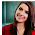# Computer System Architecture Set 25

### Questions 241 to 250

 241 Specify the Complement for the equation, F=AB+C’D’+B’D.` (a)  (A+B’)(C’+D)(B+D’)                                     (b)  (A’+B’)(C+D)(B+D’)                                     (c)  (A’+B’)(C’+D’)+(B+D’)                                (d)  (A’+B’)+(C+D)+(B’+D)                                (e)  (A’+B’)(C’+D’)(B+D’). 242 Identify the input device in which finger shows the cursor movement. (a)  Mouse                     (b)  Scanner                   (c)  Glide pad                (d)  Microphone          (e) Trackball. 243 Name the keyword to specify the number of pixels displayed on the screen. (a)  Cell                          (b)  Color depth            (c)  Monitor size           (d)  Resolution       (e)  Refresh rate. 244 Subtract the given unsigned decimal numbers by taking the 10's complement of the subtrahend.   5250 - 1321 (a)  3928                        (b)  3828                        (c)  3929                        (d)  3927                        (e)  3729. 245 From the given k-map, give the simplified equation for F. (a)  D + B'C                   (b)  D'+BC                     (c)  D + B'C'                   (d)  D + BC'                   (e)  D + BC. 246 If XY = 0 then  what is the value of XY ? (a)  X                              (b)  Y                              (c)  (X’+Y’)                    (d)  (X+Y)                      (e)  XY. 247 Specify the hexadecimal equivalent of a decimal number , 10. (a)  A                              (b)  B                              (c)  C                               (d)  D                              (e)  E. 248 Identify the 2’s complement of 101010101. (a)  010101010            (b)  101010101            (c)  010101000             (d)  110101011            (e)  010101011. 249 Name the operation represented by . (a)  OR                           (b)  AND                        (c)  XOR                         (d)  COMPLEMENT   (e)  NOR. 250 Which one of the following instructions represents  . (a)  Memory reference                                        (b)  Register reference                                          (c)  I/O instruction                                                (d)  A and C above                           (e)  None of the above.

 241 Answer :   (b) Reason:    As OR gates becomes AND gates & values change to their complements F’ = (A’+B’)(C+D)(B+D’). 242 Answer :   (c) Reason:    Glide pad is a input device for which finger shows the cursor movement. 243 Answer :   (d) Reason:    Resolution is the number of pixels displayed on the screen. 244 Answer :   (c) Reason:    10’s complement as 1321 = 86795250 + 86793929 – discard the carry. 245 Answer :   (a) Reason:    Simplify eight 1’s grouped toget D and remaining four 1’s to get B’CD + B’C. 246 Answer :   (d) Reason:    If XY = 0XY  = X + Y. 247 Answer :   (a) Reason:    The Hexadecimal equivalent of a decimal number, 10 is A. 248 Answer :   (e) Reason:    2’s complement of 101010101 is 010101011. 249 Answer :   (b) Reason:        ‘’  denotes AND. 250 Answer :   (b) Reason:    As 15th bit is 0 and Opcode 111Register reference.

29  30  31  32  33  34  35  36  37  38  39  Next >>

1.2.